# First Order Logic Propositional Logic A proposition is

• Slides: 38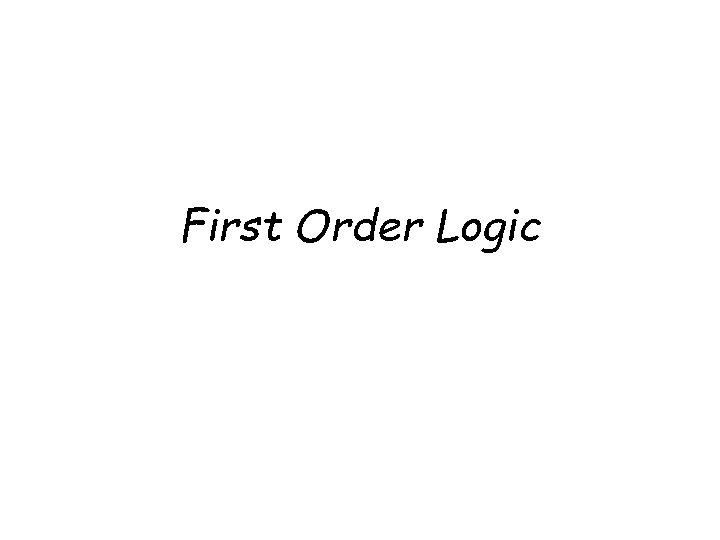First Order Logic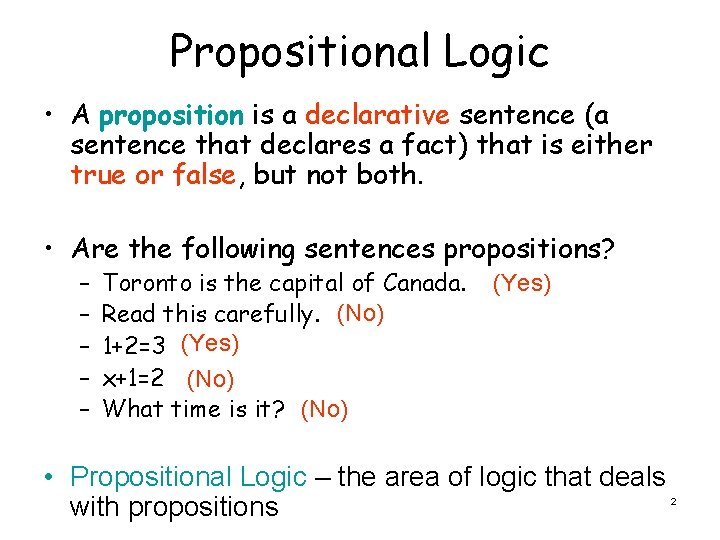Propositional Logic • A proposition is a declarative sentence (a sentence that declares a fact) that is either true or false, but not both. • Are the following sentences propositions? – – – Toronto is the capital of Canada. Read this carefully. (No) 1+2=3 (Yes) x+1=2 (No) What time is it? (No) (Yes) • Propositional Logic – the area of logic that deals with propositions 2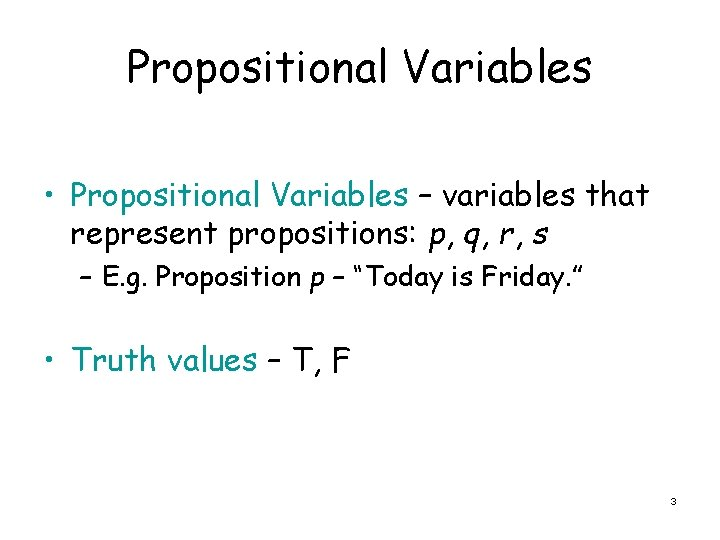Propositional Variables • Propositional Variables – variables that represent propositions: p, q, r, s – E. g. Proposition p – “Today is Friday. ” • Truth values – T, F 3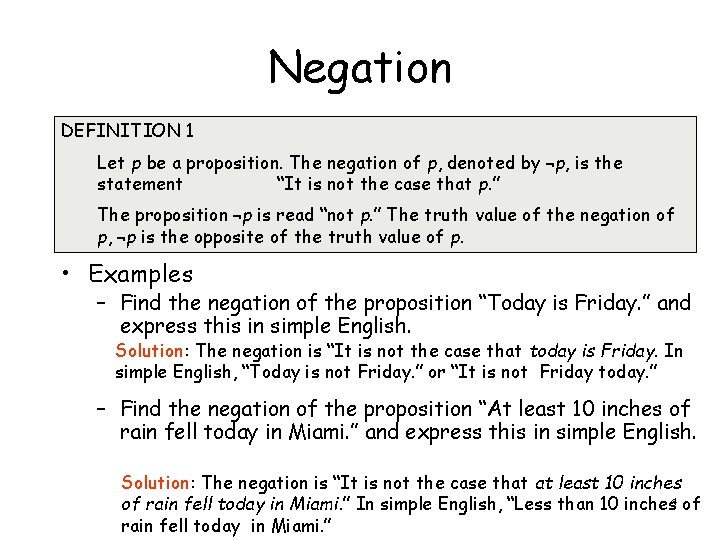Negation DEFINITION 1 Let p be a proposition. The negation of p, denoted by ¬p, is the statement “It is not the case that p. ” The proposition ¬p is read “not p. ” The truth value of the negation of p, ¬p is the opposite of the truth value of p. • Examples – Find the negation of the proposition “Today is Friday. ” and express this in simple English. Solution: The negation is “It is not the case that today is Friday. In simple English, “Today is not Friday. ” or “It is not Friday today. ” – Find the negation of the proposition “At least 10 inches of rain fell today in Miami. ” and express this in simple English. Solution: The negation is “It is not the case that at least 10 inches of rain fell today in Miami. ” In simple English, “Less than 10 inches 4 of rain fell today in Miami. ”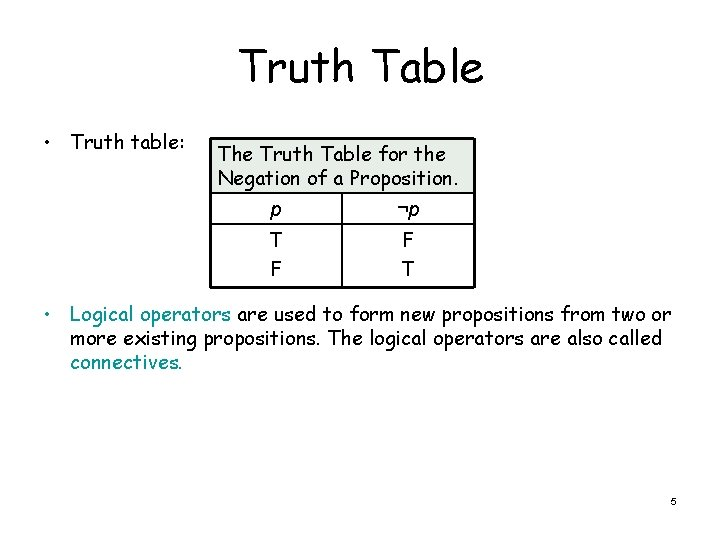Truth Table • Truth table: The Truth Table for the Negation of a Proposition. p ¬p T F F T • Logical operators are used to form new propositions from two or more existing propositions. The logical operators are also called connectives. 5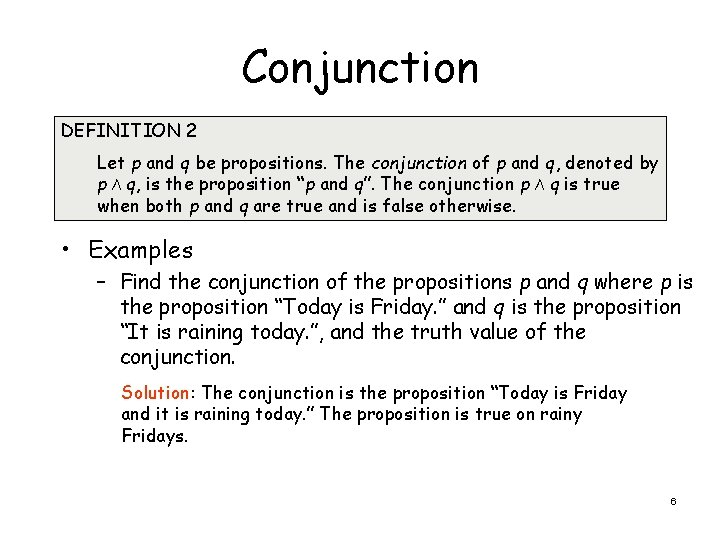Conjunction DEFINITION 2 Let p and q be propositions. The conjunction of p and q, denoted by p Λ q, is the proposition “p and q”. The conjunction p Λ q is true when both p and q are true and is false otherwise. • Examples – Find the conjunction of the propositions p and q where p is the proposition “Today is Friday. ” and q is the proposition “It is raining today. ”, and the truth value of the conjunction. Solution: The conjunction is the proposition “Today is Friday and it is raining today. ” The proposition is true on rainy Fridays. 6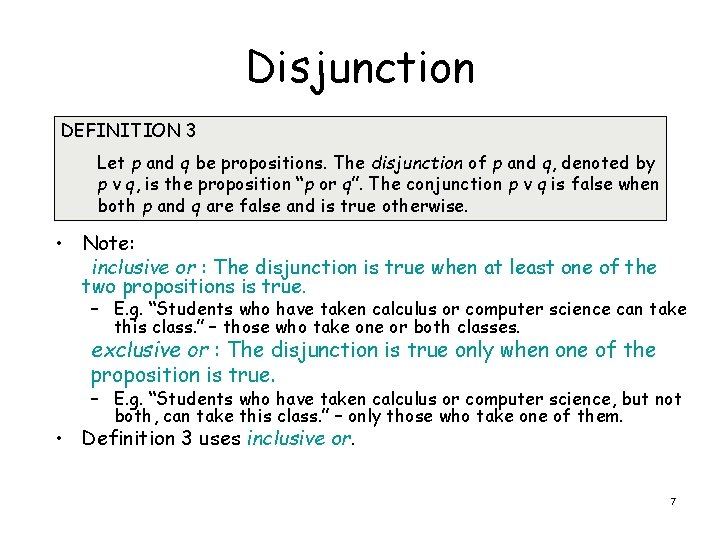Disjunction DEFINITION 3 Let p and q be propositions. The disjunction of p and q, denoted by p ν q, is the proposition “p or q”. The conjunction p ν q is false when both p and q are false and is true otherwise. • Note: inclusive or : The disjunction is true when at least one of the two propositions is true. – E. g. “Students who have taken calculus or computer science can take this class. ” – those who take one or both classes. exclusive or : The disjunction is true only when one of the proposition is true. – E. g. “Students who have taken calculus or computer science, but not both, can take this class. ” – only those who take one of them. • Definition 3 uses inclusive or. 7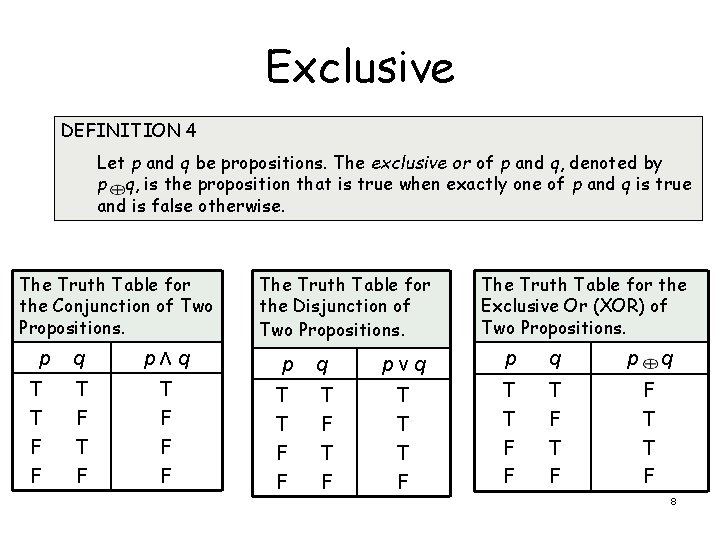Exclusive DEFINITION 4 Let p and q be propositions. The exclusive or of p and q, denoted by p q, is the proposition that is true when exactly one of p and q is true and is false otherwise. The Truth Table for the Conjunction of Two Propositions. p T T F F The Truth Table for the Disjunction of Two Propositions. q pΛq p T F T F F F T T F F q T F The Truth Table for the Exclusive Or (XOR) of Two Propositions. pνq p q T T T F F T F p q F T T F 8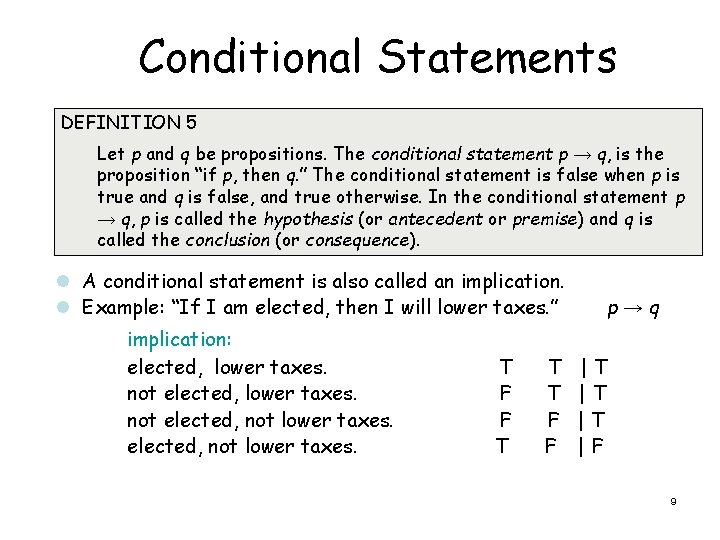Conditional Statements DEFINITION 5 Let p and q be propositions. The conditional statement p → q, is the proposition “if p, then q. ” The conditional statement is false when p is true and q is false, and true otherwise. In the conditional statement p → q, p is called the hypothesis (or antecedent or premise) and q is called the conclusion (or consequence). l A conditional statement is also called an implication. l Example: “If I am elected, then I will lower taxes. ” implication: elected, lower taxes. not elected, not lower taxes. T F F T T T F F p→q |T |T |T |F 9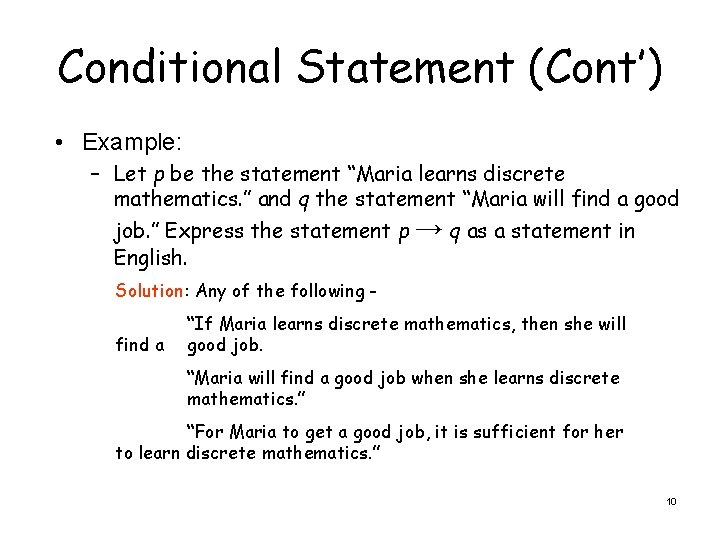Conditional Statement (Cont’) • Example: – Let p be the statement “Maria learns discrete mathematics. ” and q the statement “Maria will find a good job. ” Express the statement p → q as a statement in English. Solution: Any of the following find a “If Maria learns discrete mathematics, then she will good job. “Maria will find a good job when she learns discrete mathematics. ” “For Maria to get a good job, it is sufficient for her to learn discrete mathematics. ” 10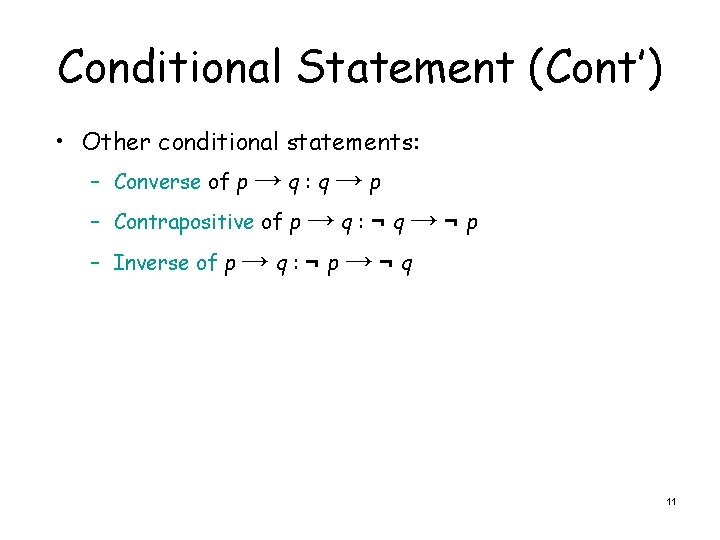Conditional Statement (Cont’) • Other conditional statements: – Converse of p → q : q → p – Contrapositive of p → q : ¬ q → ¬ p – Inverse of p → q : ¬ p → ¬ q 11Biconditional Statement DEFINITION 6 Let p and q be propositions. The biconditional statement p ↔ q is the proposition “p if and only if q. ” The biconditional statement p ↔ q is true when p and q have the same truth values, and is false otherwise. Biconditional statements are also called bi-implications. • p ↔ q has the same truth value as (p → q) Λ (q → p) • “if and only if” can be expressed by “iff” • Example: – Let p be the statement “You can take the flight” and let q be the statement “You buy a ticket. ” Then p ↔ q is the statement “You can take the flight if and only if you buy a ticket. ” Implication: If you buy a ticket you can take the flight. If you don’t buy a ticket you cannot take the flight. 12Biconditional Statement (Cont’) The Truth Table for the Biconditional p ↔ q. p T T F F q T F p↔ q T F F T 13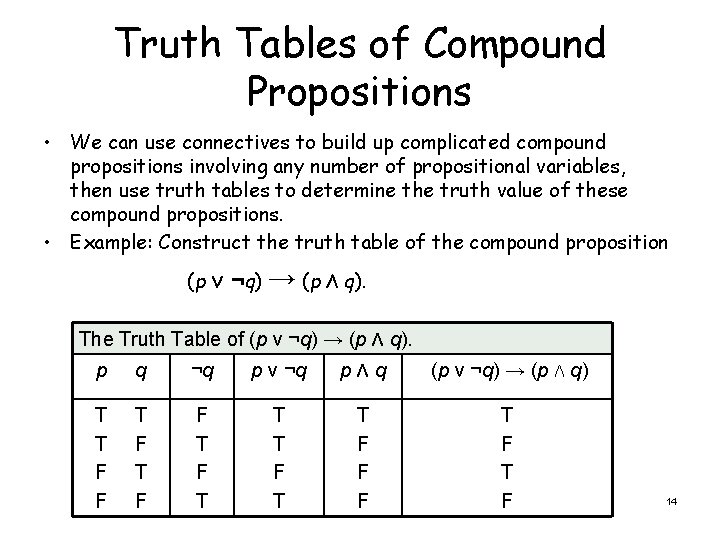Truth Tables of Compound Propositions • We can use connectives to build up complicated compound propositions involving any number of propositional variables, then use truth tables to determine the truth value of these compound propositions. • Example: Construct the truth table of the compound proposition (p ν ¬q) → (p Λ q). The Truth Table of (p ν ¬q) → (p Λ q). p q ¬q p ν ¬q pΛq (p ν ¬q) → (p Λ q) T T F F T F T T T F F F T F 14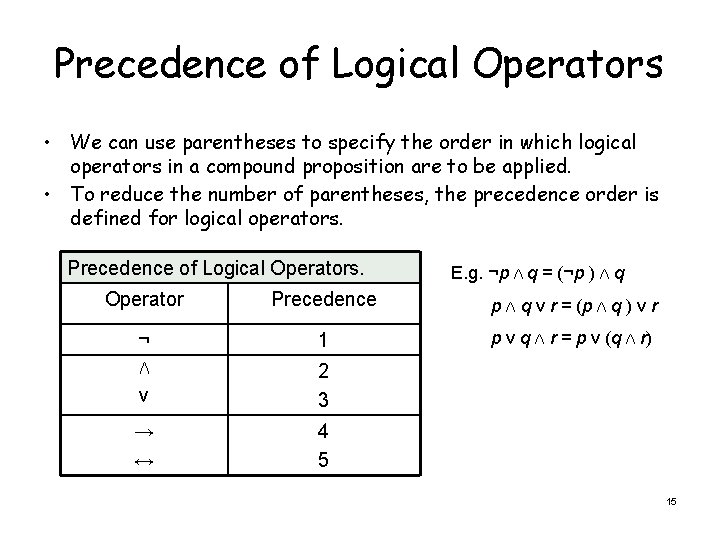Precedence of Logical Operators • We can use parentheses to specify the order in which logical operators in a compound proposition are to be applied. • To reduce the number of parentheses, the precedence order is defined for logical operators. Precedence of Logical Operators. E. g. ¬p Λ q = (¬p ) Λ q Operator Precedence p Λ q ν r = (p Λ q ) ν r ¬ 1 p ν q Λ r = p ν (q Λ r) Λ ν 2 3 → ↔ 4 5 15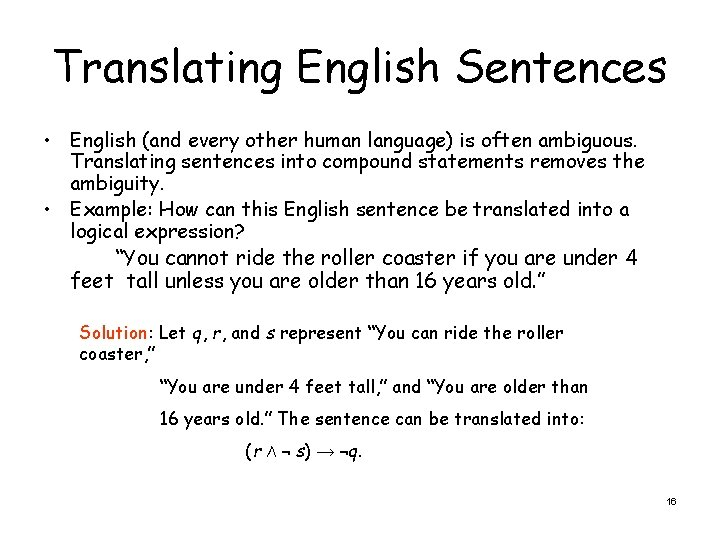Translating English Sentences • English (and every other human language) is often ambiguous. Translating sentences into compound statements removes the ambiguity. • Example: How can this English sentence be translated into a logical expression? “You cannot ride the roller coaster if you are under 4 feet tall unless you are older than 16 years old. ” Solution: Let q, r, and s represent “You can ride the roller coaster, ” “You are under 4 feet tall, ” and “You are older than 16 years old. ” The sentence can be translated into: (r Λ ¬ s) → ¬q. 16Translating English Sentences • Example: How can this English sentence be translated into a logical expression? “You can access the Internet from campus only if you are a computer science major or you are not a freshman. ” Solution: Let a, c, and f represent “You can access the Internet from campus, ” “You are a computer science major, ” and “You are a freshman. ” The sentence can be translated into: a → (c ν ¬f). 17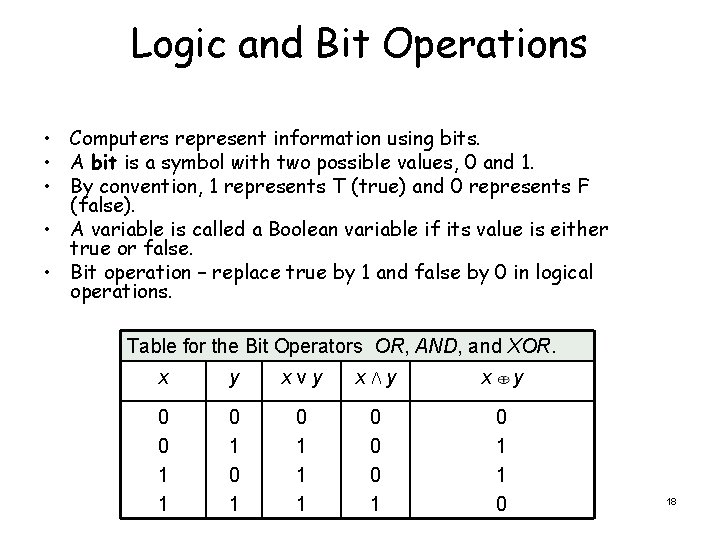Logic and Bit Operations • Computers represent information using bits. • A bit is a symbol with two possible values, 0 and 1. • By convention, 1 represents T (true) and 0 represents F (false). • A variable is called a Boolean variable if its value is either true or false. • Bit operation – replace true by 1 and false by 0 in logical operations. Table for the Bit Operators OR, AND, and XOR. x y xνy xΛy 0 0 1 1 0 1 0 1 1 1 0 0 0 1 x y 0 1 1 0 18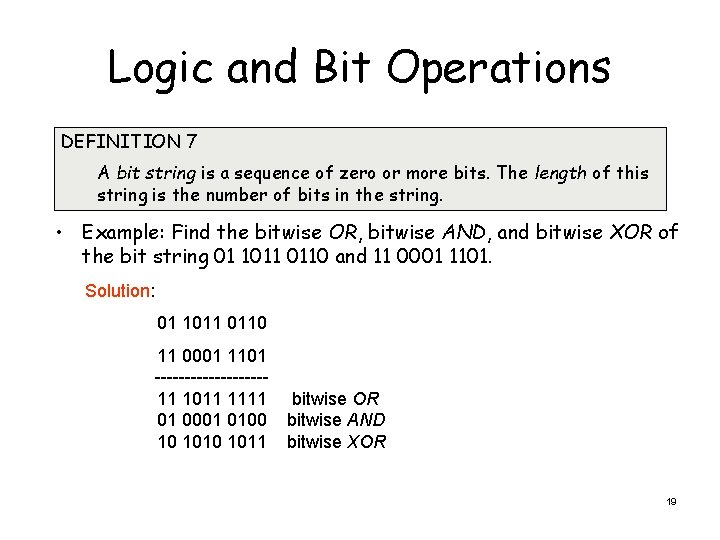Logic and Bit Operations DEFINITION 7 A bit string is a sequence of zero or more bits. The length of this string is the number of bits in the string. • Example: Find the bitwise OR, bitwise AND, and bitwise XOR of the bit string 01 1011 0110 and 11 0001 1101. Solution: 01 1011 0110 11 0001 1101 ---------11 1011 1111 01 0001 0100 10 1011 bitwise OR bitwise AND bitwise XOR 19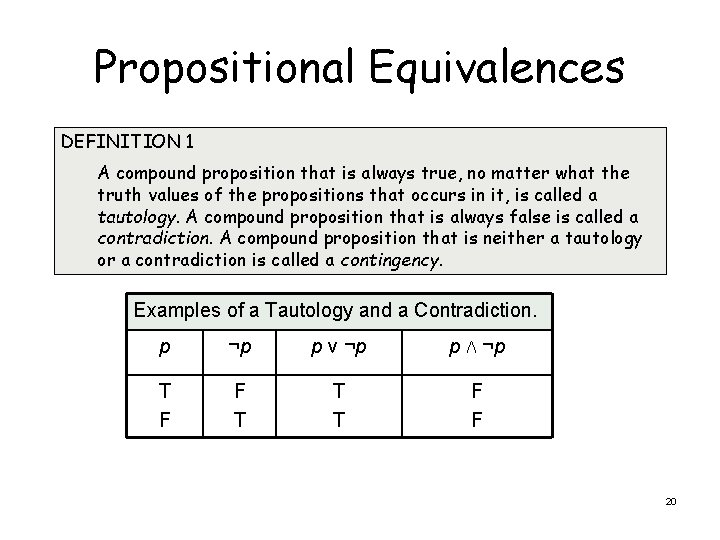Propositional Equivalences DEFINITION 1 A compound proposition that is always true, no matter what the truth values of the propositions that occurs in it, is called a tautology. A compound proposition that is always false is called a contradiction. A compound proposition that is neither a tautology or a contradiction is called a contingency. Examples of a Tautology and a Contradiction. p ¬p p ν ¬p p Λ ¬p T F F T T T F F 20Logical Equivalences DEFINITION 2 The compound propositions p and q are called logically equivalent if p ↔ q is a tautology. The notation p ≡ q denotes that p and q are logically equivalent. • Compound propositions that have the same truth values in all possible cases are called logically equivalent. • Example: Show that ¬p ν q and p → q are logically equivalent. Truth Tables for ¬p ν q and p → q. p q ¬p ¬p ν q p→q T T F F F T T T F T T 21Logical Equivalence Two statements have the same truth table De Morgan’s LawConstructing New Logical Equivalences • • • Example: Show that ¬(p → q ) and p Λ ¬q are logically equivalent. Solution: ¬(p → q ) ≡ ¬(¬p ν q) by example on earlier slide ≡ ¬(¬p) Λ ¬q by the second De Morgan law ≡ p Λ ¬q by the double negation law Example: Show that (p Λ q) → (p ν q) is a tautology. Solution: To show that this statement is a tautology, we will use logical equivalences to demonstrate that it is logically equivalent to T. (p Λ q) → (p ν q) ≡ ¬(p Λ q) ν (p ν q) by example on earlier slides ≡ (¬ p ν ¬q) ν (p ν q) by the first De Morgan law ≡ (¬ p ν p) ν (¬ q ν q) by the associative and communicative law for disjunction ≡TνT ≡T Note: The above examples can also be done using truth tables. 23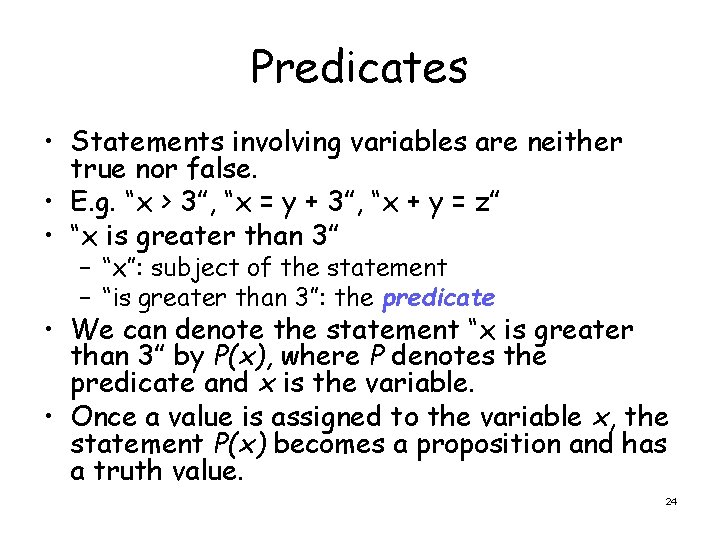Predicates • Statements involving variables are neither true nor false. • E. g. “x > 3”, “x = y + 3”, “x + y = z” • “x is greater than 3” – “x”: subject of the statement – “is greater than 3”: the predicate • We can denote the statement “x is greater than 3” by P(x), where P denotes the predicate and x is the variable. • Once a value is assigned to the variable x, the statement P(x) becomes a proposition and has a truth value. 24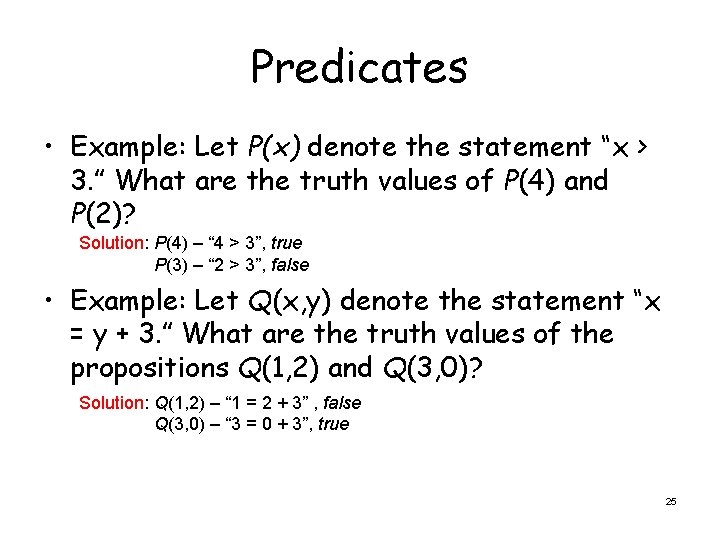Predicates • Example: Let P(x) denote the statement “x > 3. ” What are the truth values of P(4) and P(2)? Solution: P(4) – “ 4 > 3”, true P(3) – “ 2 > 3”, false • Example: Let Q(x, y) denote the statement “x = y + 3. ” What are the truth values of the propositions Q(1, 2) and Q(3, 0)? Solution: Q(1, 2) – “ 1 = 2 + 3” , false Q(3, 0) – “ 3 = 0 + 3”, true 25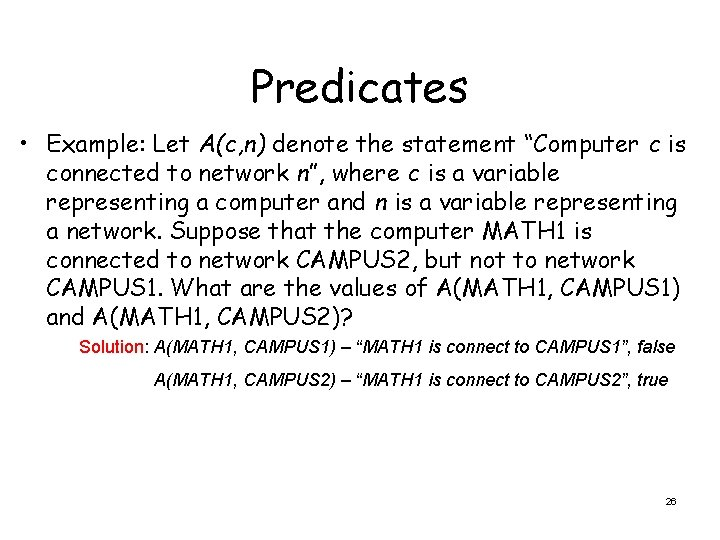Predicates • Example: Let A(c, n) denote the statement “Computer c is connected to network n”, where c is a variable representing a computer and n is a variable representing a network. Suppose that the computer MATH 1 is connected to network CAMPUS 2, but not to network CAMPUS 1. What are the values of A(MATH 1, CAMPUS 1) and A(MATH 1, CAMPUS 2)? Solution: A(MATH 1, CAMPUS 1) – “MATH 1 is connect to CAMPUS 1”, false A(MATH 1, CAMPUS 2) – “MATH 1 is connect to CAMPUS 2”, true 26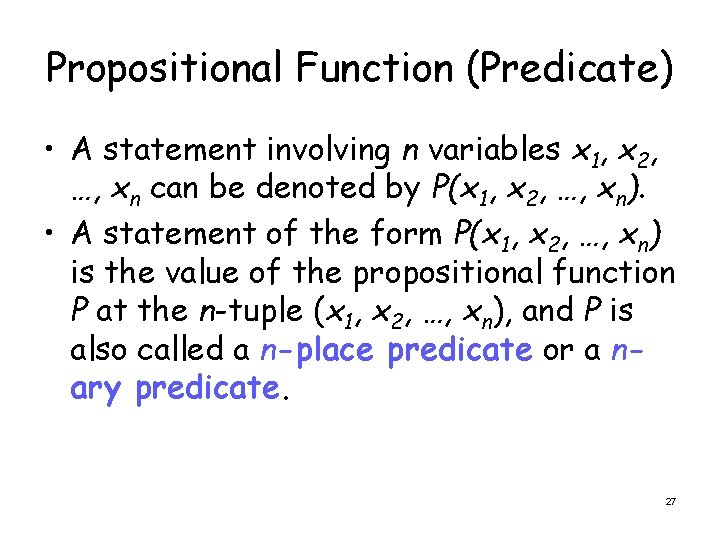Propositional Function (Predicate) • A statement involving n variables x 1, x 2, …, xn can be denoted by P(x 1, x 2, …, xn). • A statement of the form P(x 1, x 2, …, xn) is the value of the propositional function P at the n-tuple (x 1, x 2, …, xn), and P is also called a n-place predicate or a nary predicate. 27Quantifiers • Quantification: express the extent to which a predicate is true over a range of elements. • Universal quantification: a predicate is true for every element under consideration • Existential quantification: a predicate is true for one or more element under consideration • A domain must be specified. 28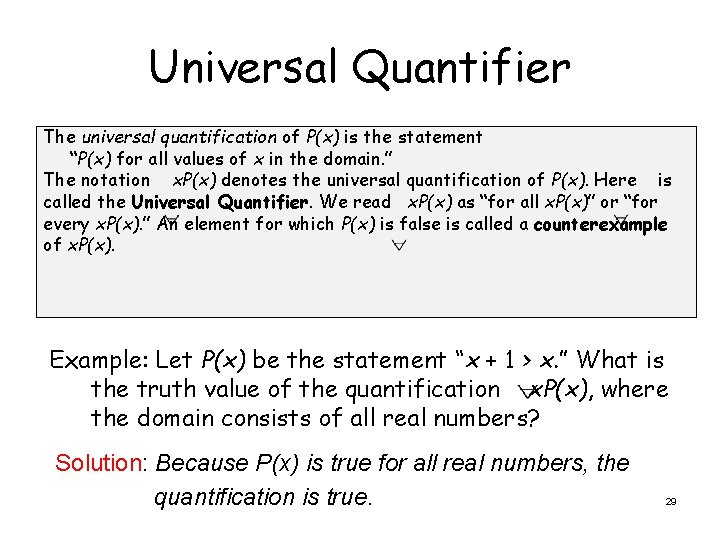Universal Quantifier The universal quantification of P(x) is the statement “P(x) for all values of x in the domain. ” The notation x. P(x) denotes the universal quantification of P(x). Here is called the Universal Quantifier. We read x. P(x) as “for all x. P(x)” or “for every x. P(x). ” An element for which P(x) is false is called a counterexample of x. P(x). Example: Let P(x) be the statement “x + 1 > x. ” What is the truth value of the quantification x. P(x), where the domain consists of all real numbers? Solution: Because P(x) is true for all real numbers, the quantification is true. 29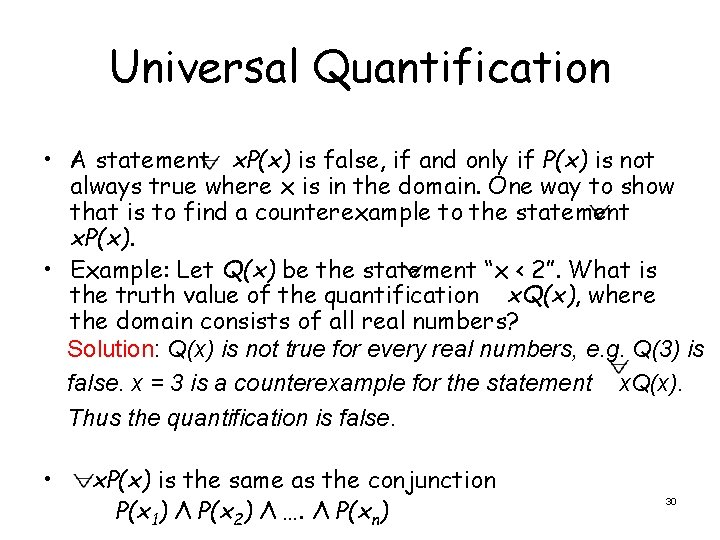Universal Quantification • A statement x. P(x) is false, if and only if P(x) is not always true where x is in the domain. One way to show that is to find a counterexample to the statement x. P(x). • Example: Let Q(x) be the statement “x < 2”. What is the truth value of the quantification x. Q(x), where the domain consists of all real numbers? Solution: Q(x) is not true for every real numbers, e. g. Q(3) is false. x = 3 is a counterexample for the statement x. Q(x). Thus the quantification is false. • x. P(x) is the same as the conjunction P(x 1) Λ P(x 2) Λ …. Λ P(xn) 30Universal Quantifier • Example: What does the statement x. N(x) mean if N(x) is “Computer x is connected to the network” and the domain consists of all computers on campus? Solution: “Every computer on campus is connected to the network. ” 31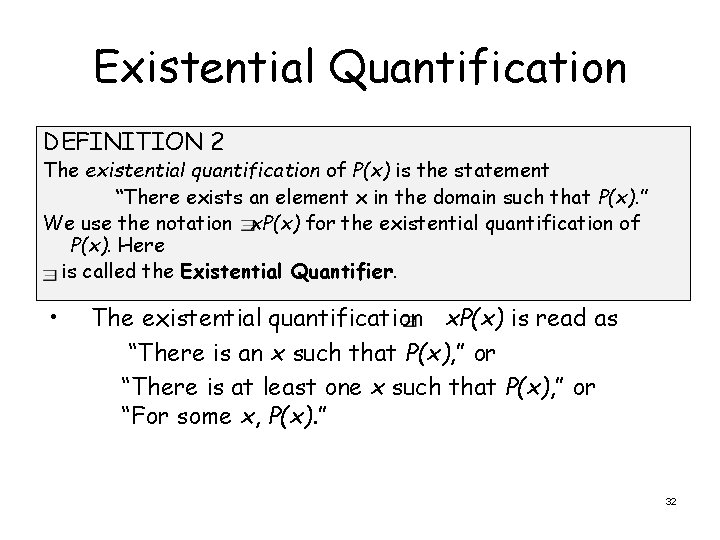Existential Quantification DEFINITION 2 The existential quantification of P(x) is the statement “There exists an element x in the domain such that P(x). ” We use the notation x. P(x) for the existential quantification of P(x). Here is called the Existential Quantifier. • The existential quantification x. P(x) is read as “There is an x such that P(x), ” or “There is at least one x such that P(x), ” or “For some x, P(x). ” 32Existential Quantification • Example: Let P(x) denote the statement “x > 3”. What is the truth value of the quantification x. P(x), where the domain consists of all real numbers? Solution: “x > 3” is sometimes true – for instance when x = 4. The existential quantification is true. • x. P(x) is false if and only if P(x) is false for every element of the domain. • Example: Let Q(x) denote the statement “x = x + 1”. What is the true value of the quantification x. Q(x), where the domain consists for all real numbers? Solution: Q(x) is false for every real number. The existential 33 quantification is false.Existential Quantification • If the domain is empty, x. Q(x) is false because there can be no element in the domain for which Q(x) is true. • The existential quantification x. P(x) is the same as the disjunction P(x 1) V P(x 2) V … VP(xn) Quantifiers Statement When True? x. P(x) is true for every x. P(x) There is an x for which P(x) is true. When False? There is an x for which x. P(x) is false for every x. 34Uniqueness Quantifier • Uniqueness quantifier ! or – !x. P(x) or true. ” 1 P(x) 1 states “There exists a unique x such that P(x) is • Quantifiers with restricted domains – Example: What do the following statements mean? The domain in each case consists of real numbers. • x < 0 (x 2 > 0): For every real number x with x < 0, x 2 > 0. “The square of a negative real number is positive. ” It’s the same as x(x < 0 → x 2 > 0) • y ≠ 0 (y 3 ≠ 0 ): For every real number y with y ≠ 0, y 3 ≠ 0. “The cube of every nonzero real number is non-zero. ” It’s the same as y(y ≠ 0 → y 3 ≠ 0 ). • z > 0 (z 2 = 2): There exists a real number z with z > 0, such that z 2 = 2. “There is a positive square root of 2. ” It’s the same as z(z > 0 Λ z 2 = 2): 35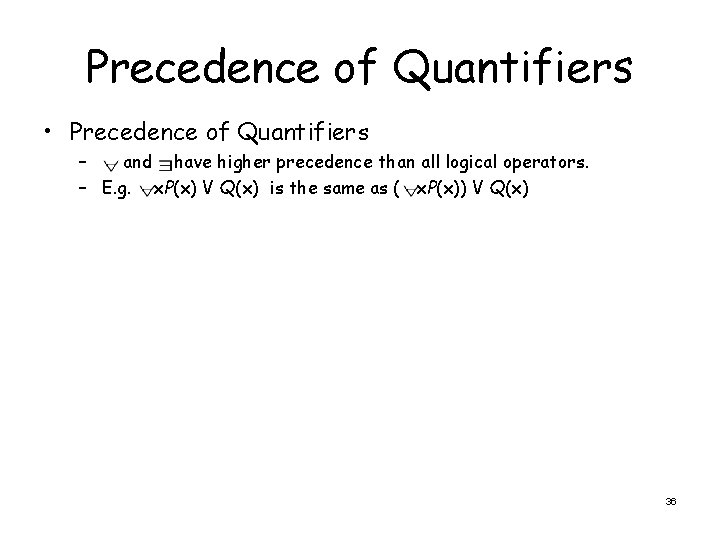Precedence of Quantifiers • Precedence of Quantifiers – and have higher precedence than all logical operators. – E. g. x. P(x) V Q(x) is the same as ( x. P(x)) V Q(x) 36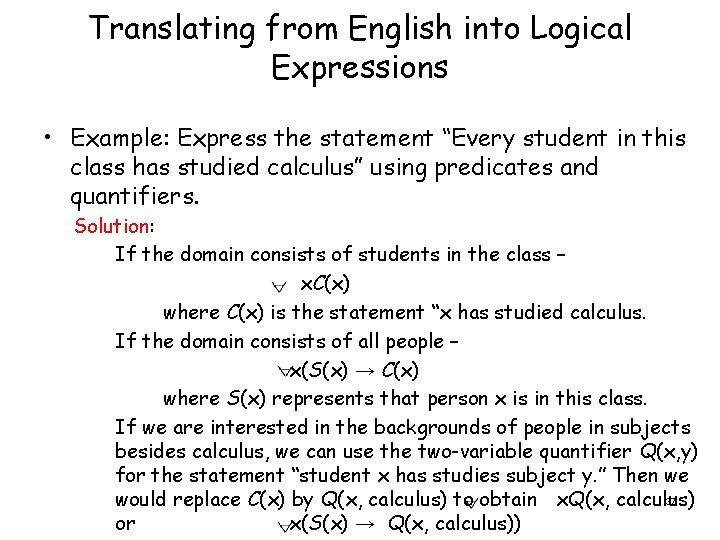Translating from English into Logical Expressions • Example: Express the statement “Every student in this class has studied calculus” using predicates and quantifiers. Solution: If the domain consists of students in the class – x. C(x) where C(x) is the statement “x has studied calculus. If the domain consists of all people – x(S(x) → C(x) where S(x) represents that person x is in this class. If we are interested in the backgrounds of people in subjects besides calculus, we can use the two-variable quantifier Q(x, y) for the statement “student x has studies subject y. ” Then we 37 would replace C(x) by Q(x, calculus) to obtain x. Q(x, calculus) or x(S(x) → Q(x, calculus))Translating from English into Logical Expressions • Example: Consider these statements. The first two are called premises and the third is called the conclusion. The entire set is called an argument. “All lions are fierce. ” “Some lions do not drink coffee. ” “Some fierce creatures do not drink coffee. ” Solution: Let P(x) be “x is a lion. ” Q(x) be “x is fierce. ” R(x) be “x drinks coffee. ” x(P(x) → Q(x)) x(P(x) Λ ¬R(x)) x(Q(x) Λ ¬R(x)) 38